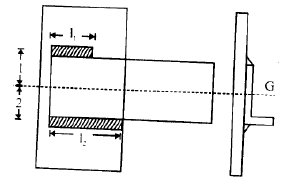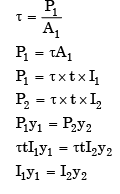Courses

# Design Of Joint - MCQ Test 2

## 30 Questions MCQ Test Mock Test Series - Mechanical Engineering (ME) for GATE 2020 | Design Of Joint - MCQ Test 2

Description
This mock test of Design Of Joint - MCQ Test 2 for Mechanical Engineering helps you for every Mechanical Engineering entrance exam. This contains 30 Multiple Choice Questions for Mechanical Engineering Design Of Joint - MCQ Test 2 (mcq) to study with solutions a complete question bank. The solved questions answers in this Design Of Joint - MCQ Test 2 quiz give you a good mix of easy questions and tough questions. Mechanical Engineering students definitely take this Design Of Joint - MCQ Test 2 exercise for a better result in the exam. You can find other Design Of Joint - MCQ Test 2 extra questions, long questions & short questions for Mechanical Engineering on EduRev as well by searching above.
QUESTION: 1

### A circular rod of diameter d is welded to a flat plate along its circumference by fillet weld of thickness t. Assuming τw as the allowable shear stress for the weld material, what is the value of the safe torque that can be transmitted?

Solution: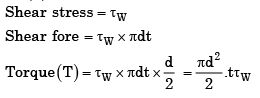QUESTION: 2

### A circular solid rod of diameter d welded to a rigid flat plate by a circular fillet weld of throat thickness t is subjected to a twisting moment T. The maximum shear stress induced in the weld is

Solution: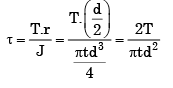QUESTION: 3

### The permissible stress in a filled weld is 100 N/mm2. The fillet weld has equal leg lengths of 15 mm each. The allowable shearing load on weldment per cm length of the weld is

Solution:

Load allowed = 100 x 0.707 x 10 x15 = 10.6 kN

QUESTION: 4

Consider the case of a squarethreaded screw loaded by a nut as shown in the given figure. The value of the average shearing stress of the screw is given by (symbols have the usual meaning)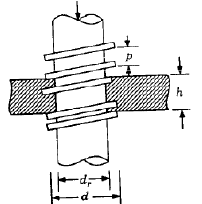Solution:
QUESTION: 5

Assertion (A): Uniform-strength bolts are used for resisting impact loads.

Solution:

A is true and R is false

QUESTION: 6

How can shock absorbing capacity of a bolt be increased?

Solution:
QUESTION: 7

The number of slots is a 25 mm castle nut is

Solution:
QUESTION: 8

A key connecting a flange coupling to a shaft is likely to fail in

Solution:

Shear is the dominant stress on the key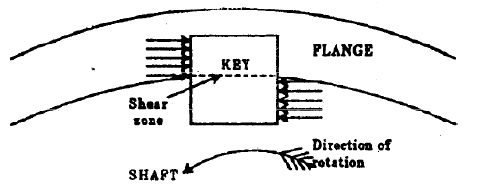QUESTION: 9

Match List I with List II and select the correct answer using the code given below the Lists: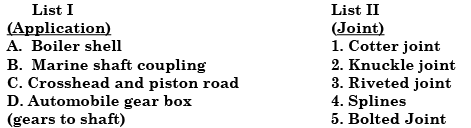Solution:
QUESTION: 10

Match List I with List II and select the correct answer using the code given below the Lists: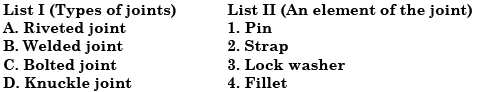codes A B C D

Solution:
QUESTION: 11

Match List I (Items in joints) with List II (Type of failure) and select the correct answer using the codes given below the Lists: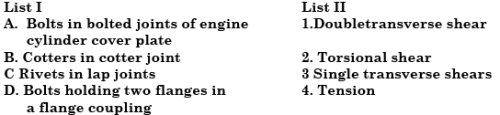Codes A B C D

Solution:
QUESTION: 12

The spigot of a cotter joint has a diameter D and carries a slot for cotter. The permissible crushing stress is x times the permissible tensile stress for the material of spigot where x > 1. The joint carries an axial load P. Which one of the following equations will give the diameter of the spigot?

Solution:
QUESTION: 13

The piston rod and the crosshead in a steam engine are usually connected by means of

Solution:
QUESTION: 14

Match List I with List II and select the correct answer using the codes given below the lists: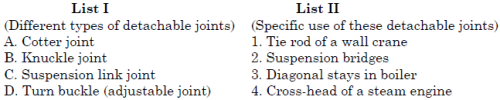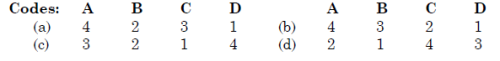Solution:
QUESTION: 15

Match List I with List II and select the correct answer using the codes given below the lists:

List I (Type of joint)
A.Cotter joint
B.Knuckle joint
C.Turn buckle
D.Riveted joint

List II (Mode of jointing members)
1.Connects two rods or bars permitting small amount of flexibility
2.Rigidly connects two members
3.Connects two rods having threaded ends
4.Permanent fluid-tight joint between two flat pieces
5.Connects two shafts and transmits torque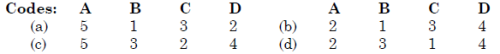Solution:

A cotter is a flat wedge-shaped piece of steel. This is used to connect rigidly two rods which transmit motion in the axial direction, without rotation. These joints may be subjected to tensile or compressive forces along the axes of the rods. Connection of piston rod to the cross-head of a steam engine, valve rod and its stem etc are examples of cotter joint.

QUESTION: 16

In the assembly of pulley, key and shaft

Solution:

Key is made the weakest so that it is cheap and easy to replace in case of failure.

QUESTION: 17

Match List-I (Type of keys) with List-II (Characteristic) and select the correct answer using the codes given below the Lists: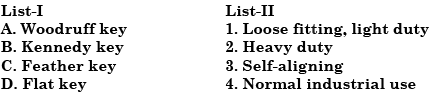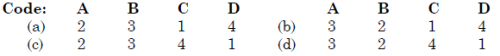Solution:

A feather key is used when one component slides over another. The key may be fastened either to the hub or the shaft and the kayway usually has a sliding fit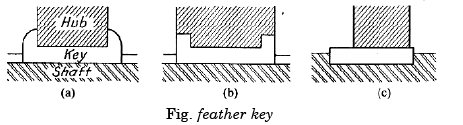QUESTION: 18

Assertion (A): The effect of keyways on a shaft is to reduce its load carrying capacity and to increase its torsional rigidity.

Reason (R): Highly localized stresses occur at or near the corners of keyways.

Solution:
QUESTION: 19

What is sunk key made in the form of a segment of a circular disc of uniform thickness, known as?

Solution:
QUESTION: 20

What are the key functions of a master schedule?

1. To generate material and capacity requirements

2. To maintain valid priorities

3. An effective capacity utilization

4. Planning the quantity and timing of output over the intermediate time horizons

Select the correct answer using the code given below:

Solution:
QUESTION: 21

Which one of the following statements is correct?

While designing a parallel sunk key it is assumed that the distribution of force along the length of the key

Solution:

Parallel sunk key. The parallel sunk keys may be of rectangular or square section uniform in width and thickness throughout. It may be noted that a parallel key is a taperless and is used where the pulley, gear or other mating piece is required to slide along the shaft. In designing a key, forces due to fit of the key are neglected and it is assumed that the distribution of forces along the length of key is uniform.

QUESTION: 22

Match List-I (Device) with List-II (Component/Accessory) and select the correct answer using the codes given below the Lists: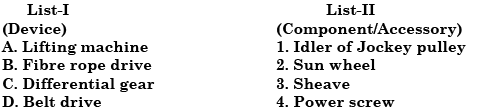[IES 2003]

Solution:
QUESTION: 23

Assertion (A): A Woodruff key is an easily adjustable key.
Reason (R): The Woodruff key accommodates itself to any taper in the hub or boss of the mating piece.

Solution: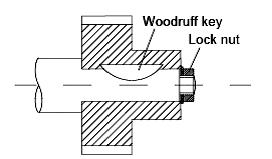The main advantages of a woodruff key are as follows:
1.It accommodates itself to any taper in the hub or boss of the mating piece.
2.It is useful on tapering shaft ends. Its extra depth in the shaft prevents any tendency to turn over in its keyway.
The main dis-advantages of a woodruff key are as follows:
1.The depth of the keyway weakens the shaft.
2.It can not be used as a feather.

QUESTION: 24

Match List I (Keys) with List II (Characteristics) and select the correct answer using the codes given below the Lists: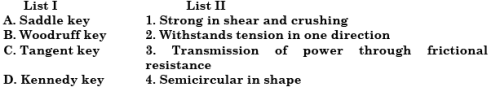Codes:  A B C D

Solution:
QUESTION: 25

Match List-I with List-II and select the correct answer using the code given below the Lists: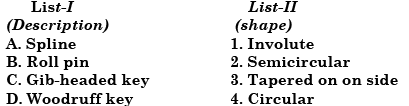Codes: A B C D

Solution:
QUESTION: 26

Consider the following statements:

A splined shaft is used for

1. Transmitting power

2. Holding a flywheel rigidly in position

3. Moving axially the gear wheels mounted on it

4. Mounting V-belt pulleys on it.

Of these statements

Solution:
QUESTION: 27

A single parallel fillet weld of total length L and weld size h subjected to a tensile load P, will have what design stress?

Solution: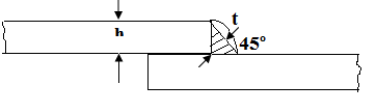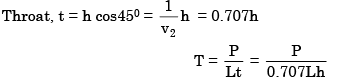QUESTION: 28

A butt welded joint, subjected to tensile force P is shown in the given figure, l = length of the weld (in mm) h = throat of the butt weld (in mm) and H is the total height of weld including reinforcement. The average tensile stress σt, in the weld is given by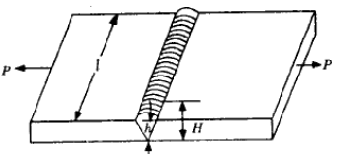Solution:
QUESTION: 29

A double fillet welded joint with parallel fillet weld of length L and leg B is subjected to a tensile force P. Assuming uniform stress distribution, the shear stress in the weld is given by

Solution:
QUESTION: 30

Assertion (A): In design of double fillet welding of un symmetrical sections with plates subjected to axial loads lengths of parallel welds are made unequal.

Reason (R): The lengths of parallel welds in fillet welding of an un symmetrical section with a plate are so proportioned that the sum of the resisting moments of welds about the centre of gravity axis is zero.]

Solution: